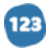# Scientific Analysis on Enthaply Vaporization of Water Lab

explanatory Essay
749 words
749 wordsThere are many concepts explored throughout “Enthalpy Vaporization of Water”. First of all, the purpose of this lab was to determine the water’s vapor pressure at different temperatures as well as to measure the molar heat of vaporization of water using the Clausias Clapeyron equation. The first concept out of many represented in this lab is the ideal gas law. The ideal gas law is used to get the number of moles of air trapped in the 10 mL graduated cylinder. Once we cooled the system so that water vapor is extremely minute, and then we determined the number of moles of air using the ideal gas law. The number of moles of air equals to the pressure (in atm) times volume divided by constant times temperature. One would assume that when the water is heated to 80 degrees, the number of air molecules in the air bubble would decrease, but it actually stays constant. This is due to the fact that there is no air coming in or out of the cylinder. As the temperature gets closer to 80 degrees, the number of air molecules stays the same but the water vapor increases. And the bubble expands to keep the pressure at the same level. The ideal gas law was also used when the partial pressure of air in the gas mixture is calculated. This is gotten from number of moles multiplied by the constant and the constant and the whole thing divided by the volume. Next Dalton’s law of partial pressure is used. The mixture of gas in the graduated cylinder was filled with two things: water vapor and air. Using the Dalton’s law, it can be concluded that the total pressure is equal to the pressure of air and the pressure of water vapor added together. This is an endothermic reaction which means that it absorbs heat, and when a reaction gains heat, it is repres... ... middle of paper ... ...inty between 1.0% (0.1/10.00*100) and 2.13% in the measured volume and 0.1/4.70*100). We also used a digital thermometer that allowed us to read the temperature readings from five degrees celcius to eighty degrees celcius. Since the digital thermometer have an absolute accuracy of plus or minus one degree celcius, it gives a percent uncertainty between 0.125 % (0.1 / 5.00 * 100) and 0.2 % (0.1/ 80.0 * 100). One of the difficulties we faced during the lab is reading the inverted graduated cylinder. To account for the inverse meniscus, we subtracted 0.2 mL from all the volumetric measurements to account for that. Volumetric uncertainty is the most important in determining the accuracy of this experiment since we are constantly checking for the volume throughout the lab. It also is the factor that gives the highest percent uncertainty out of all the instruments used.

#### In this essay, the author

• Explains the ideal gas law, which is used to determine the water's vapor pressure at different temperatures, and the molar heat of vapourization using the clausias clapeyron equation.
• Explains how dalton's law of partial pressure is applied to the mixture of gas in the graduated cylinder filled with water vapor and air.
• Explains that the enthalpy of vaporization is dependent on the strength of the intermolecular gases. the clausius clapeyron equation shows the correlation between a liquid and the temperature of that same liquid.

•